# 31 Year NEET Previous Year Questions: Units & Measurement

## 40 Questions MCQ Test Topic-wise MCQ Tests for NEET | 31 Year NEET Previous Year Questions: Units & Measurement

Description
Attempt 31 Year NEET Previous Year Questions: Units & Measurement | 40 questions in 80 minutes | Mock test for NEET preparation | Free important questions MCQ to study Topic-wise MCQ Tests for NEET for NEET Exam | Download free PDF with solutions
QUESTION: 1

### The dimensional formula for angular momentum is  

Solution:

[Angular momentum ] = [Momentum of inertia] × [Angular velocity] = ML2 × T–1
= ML2T–1

OR

In three dimensions, the angular momentum for a point particle is a pseudovector r × p, the cross product of the particle's position vector r (relative to some origin) and its momentum vector; the latter is p = mv in Newtonian mechanics.

In SI base units: kg m2 s−1

Dimension: M L2T−1

Derivations from other quantities: L = Iω = r × p

QUESTION: 2

### If C and R denote capacitance and resistance, the dimensional formula of CR is 

Solution:

CR = [M -1 L-2 T 4 A 2 ][M1 L2 T -3 A -2 ] = [T] = [M0 L0 T1]

QUESTION: 3

### The dimensional formula of torque is 

Solution:

ζ = [Force × distance] = [MLT–2] [L] = ML2T–2

QUESTION: 4

Dimensional formula of self inductance is 

Solution:

Induced emf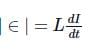where L is the self inductance and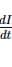is the rate of change of current.

∴ Dimensional formula of  L =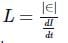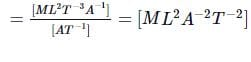QUESTION: 5

Of the following quantities, which one has dimension different from the remaining three? 

Solution:

For angular momentum, the dimensional formula is ML2T–1. For other three, it is ML-1T–2.

QUESTION: 6

If x = at + bt2, where x is the distance travelled by the body in kilometers while t is the time in seconds, then the units of b is 

Solution: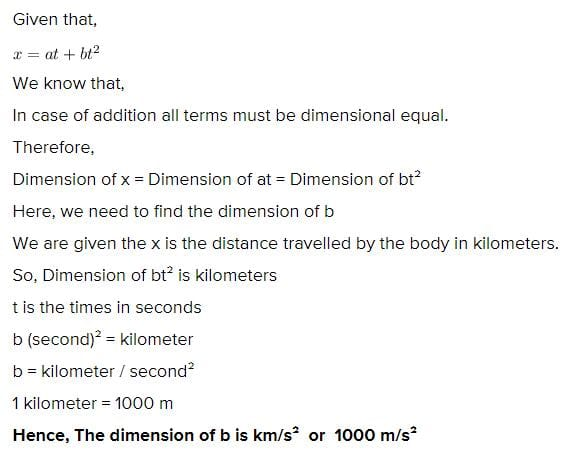QUESTION: 7

According to Newton, the viscous force acting between liquid layers of area A and velocity gradient ΔV/ΔZ is given by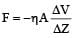where η is constant called coefficient of viscosity. The dimensional formula of η is 

Solution:

Substitute the dimension al formula of F, A, ΔV and ΔZ on both sides and find that for η.

QUESTION: 8

The frequency of vibration f of a mass m suspended from a spring of spring constant k is given by a relation of the type f = c mx ky, where c is a dimensionless constant. The values of x and y are

Solution:

f = c mx ky; Spring constant k = force/length. [M0L0T–1] = [Mx (MT–2)y] =[ Mx + y T–2y]

⇒ x+y = 0, -2y = -1 or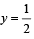Therefore,  x = -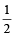QUESTION: 9

The dimensional formula of pressure is 

Solution:

[Pressure] = [Force] / [Area]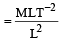= ML–1T–2

QUESTION: 10

The dimension al formula for permeability µ is given by 

Solution: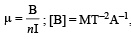[n] = L–1,  [I] = A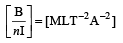QUESTION: 11

A certain body weighs 22.42 gm and has a measured volume of 4.7 cc. The possible error in the measurement of mass and volume are 0.01 gm and 0.1 cc. Then maximum error in the den sity will be 

Solution:

D = M/V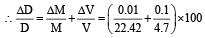=2 %

QUESTION: 12

P represents radiation pressure, crepresents speed of light and S represents radiation energy striking unit area per sec. The non zero integers x, y, z such that Px Sy cz is dimensionless are 

Solution:

Try out the given alter natives.
When x = 1, y = –1, z = 1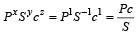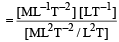= [M0L0T0]

QUESTION: 13

The time dependence of a physical quantity p is given by p = p0 exp (– αt2), where α is a constant and t is the time. The constant α 

Solution:

In p = p0 exp (–αt2), αt2 dimension less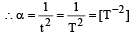QUESTION: 14

urpentine oil is flowing through a tube of length l and radius r. The pressure difference between the two ends of the tube is P. The viscosity of oil is given by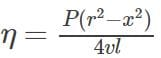where v is the velocity of oil at a distance a from the axis of the tube. The dimensions of η are

Solution: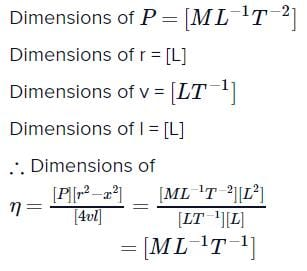QUESTION: 15

In a vernier calliper N divisions of vernier scale coincides with (N – 1) divisions of main scale (in which length of one division is 1 mm). The least count of the instrument should be

Solution:

Least count = 1MSD – 1 VSD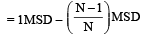(∵ N  VSD = (N – 1)MSD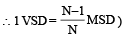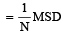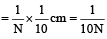QUESTION: 16

In a particular system, the unit of length, mass and time are chosen to be 10 cm, 10 g and 0.1 s respectively. The unit of force in this system will be equivalen t to 

Solution:

[F] = MLT–2 = (10g) (10 cm) (0.1s)–2
= (10–2 kg) (10–1m) (10–1s)–2 = 10–1N.

QUESTION: 17

Which of the following is a dimensional constant? 

Solution:

A quantity which has dimensions and a constant value is called dimensional constant. Therefore, gravitational constant (G) is a dimensional constant.

QUESTION: 18

The percentage errors in the measurement of mass and speed are 2% and 3% respectively.The error in kinetic energy obtained by measuring mass and speed will be 

Solution:

Percentage error in mass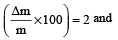percentage error in speed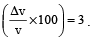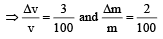Kinetic energy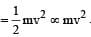∴ Error in measurement of kinetic energy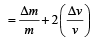By Binomial Function ,

Reqd. error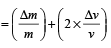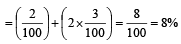∴ %age error = 8%.

QUESTION: 19

An equation is given as :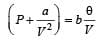where P = Pressure, V = Volume & θ = Absolute temperature. If a and b are constants, then dimensions of a will be 

Solution: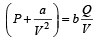According to the principle of homogeinity quantity with same dimension can be added or subtracted.
Hence, Dimension of P = Dimension of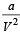⇒ Dimension of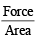= Dimension of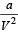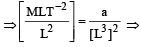a = [M L5 T–2]

QUESTION: 20

The density of a cube is measured by measuring its mass and length of its sides. If the maximum error in the measurement of mass and length are 4% and 3% respectively, the maximum error in the measurement of den sity will be 

Solution:

Density =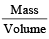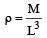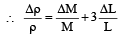% error in density = % error in Mass + 3 (% error in length]
= 4 + 3(3) = 13%

QUESTION: 21

Which of the following will have the dimensions of time           

Solution: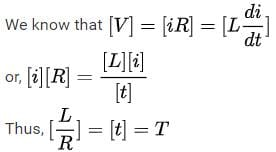QUESTION: 22

The force F on a sphere of radius  a moving in a medium with velocity v is  given by F = 6πηav.The dimensions of η are 

Solution:

F= 6πη av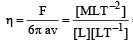= ML–1T–1

QUESTION: 23

The dimensional formula for magnetic flux is 

Solution:

Dimension of magnetic flux = Dimension of voltage × Dimension of time = [ML2T–3A–1] [T]  = [ML2T–2A–1]

∵ Voltage =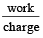QUESTION: 24

Which one of the following groups have quantities that do not have the same dimensions? 

Solution:

Force has dimension [MLT–2] while impulse has dimension [MLT–1], both have different dimensions.

QUESTION: 25

The dimensions of Planck’s constant are same as    

Solution:

We know that E = hv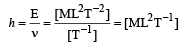Angular momentum = Iω
= [ML2][T–1] = [ML2T–1]

QUESTION: 26

The unit of the Stefan-Boltzmann's constant is   

Solution:

E = σAT4
E is energy dissipated per second.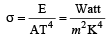QUESTION: 27

The unit of permittivity of free space, εo is  

Solution: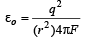⇒ unit of εo is (coulomb)2/ newton -metre2

QUESTION: 28

The dimensions of universal gravitational constant are [1992, 2004]

Solution: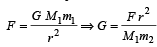∴  dimension of G is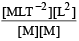= M–1L3T–2

QUESTION: 29

The ratio of the dimension of Planck’s constant and that of the moment of inertia has the dimension of 

Solution: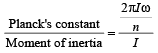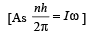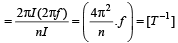QUESTION: 30

The velocity v of a particle at time t is given by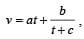where a, b and c are constant.The dimensions of a, b and c are respectively 

Solution:

Dimension of  a. t = dimension of velocity a . t = LT-1 ⇒ a = LT-2
Dimension of c = dimension of t (two physical quantity of same dimension can only be added)

So, dimension of c = T

Dimension of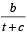= Dimension of v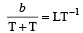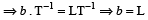So, answer is LT–2, L & T

QUESTION: 31

Dimensions of resistance in an electrical circuit, in terms of dimension of mass M, of length L, of time T and of current I, would be 

Solution:

Dimensions of Resistance,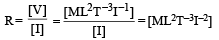QUESTION: 32

If the error in the measurement of radius of a sphere is 2%, then the error in the determination of volume of the sphere will be: 

Solution:

Error in the measurement of radius of a sphere = 2%

Volume of the sphere =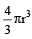∴  Error in the volume =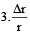= 3 × 2% = 6%

QUESTION: 33

Which two of the following five physical parameters have the same dimensions?
(A) Energy density 
(B) Refractive index
(C) Dielectric constant
(D) Young’s modulus
(E) Magnetic field

Solution:

[Energy density] =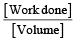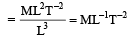[Young’s Modulus] =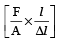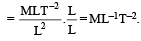QUESTION: 34

If the dimensions of a physical quantity are given by Ma Lb Tc, then the physical quantity will be:

Solution:
QUESTION: 35

The dimension ofwhere ε0 is permittivity of free space and E is electric field, is:

Solution: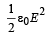represents energy density i.e., energy per unit volume.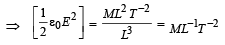QUESTION: 36

The dimensions of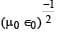are    

Solution: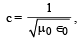, so, dimensions are [LTT–1].

QUESTION: 37

The density of material in CGS system of units is 4g/cm3. In a system of units in which unit of length is 10 cm and unit of mass is 100 g, the value of density of material will be [2011M]

Solution:

In CGS system,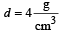The unit of mass is 100g and unit of length is 10 cm, so

density =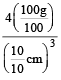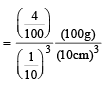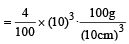= 40 unit

QUESTION: 38

The dimensions of (μ0ε0)–1/2 are : [2012M]

Solution: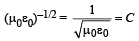: speed of light

where ε0 =  permittivity of free space

μ0 = permeability of free space

So dimension LT–1

QUESTION: 39

In an experiment four quantities a, b, c and d are measured with percentage error 1%, 2%, 3% and 4% respectively. Quantity P is calculated as follows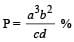error in P is [NEET 2013]

Solution: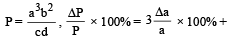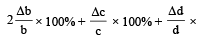100%.
= 3 × 1% + 2 × 2% + 3% + 4% = 14%

QUESTION: 40

The pair of quantities having same dimensions is [NEET Kar. 2013]

Solution:

Work = Force ×  displacement Torque = Force × force arm

= mass × acceleration × length

= [M] × [LT–2] × [L] = [M L2T–2]Use Code STAYHOME200 and get INR 200 additional OFF Use Coupon Code